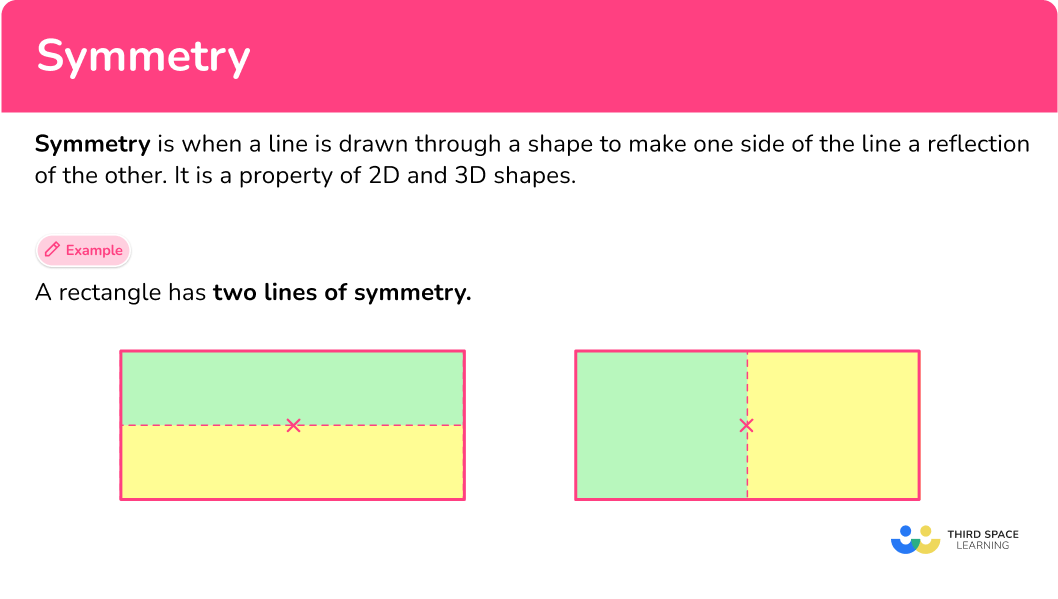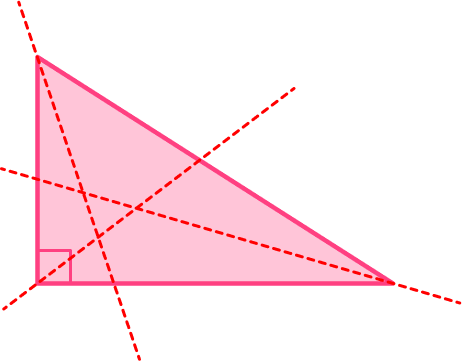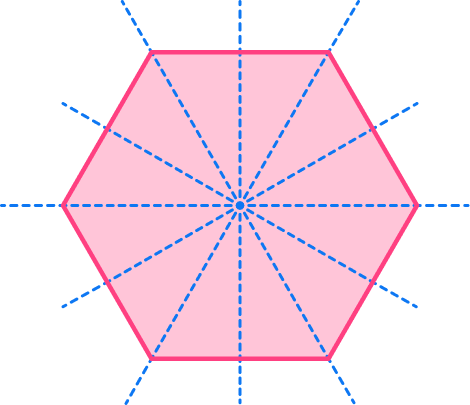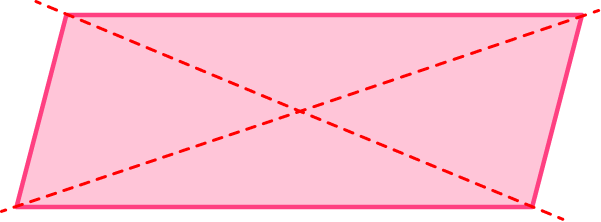# Symmetry

Here you will learn about symmetry, including the definition of symmetry, how to recognize a line of symmetry in 2D shapes, and how to identify line of symmetry in figures.

Students will first learn about symmetry as a part of geometry in 4th grade.

## What is symmetry?

Symmetry is when a line is drawn through a shape to make one side of the line a reflection of the other.

Symmetry is a property of 2D and 3D geometrical shapes and objects that divides them into two identical halves that mirror, or are mirror images of each other.

When an imaginary line is drawn through a shape to make one side of the line a true reflection of the other (and vice versa), the shape is known as symmetrical.

Examples of objects in the real world that are symmetrical include snowflakes, the feathers of peacocks, and the wings of butterflies.

A shape has asymmetry if both sides are not the same when cut in half by an imaginary line. These shapes are called asymmetric.

Examples of objects in the real world that are asymmetrical include trees, some leaves, and the fiddler crab.

### Lines of symmetry

An axis of symmetry is an imaginary line that splits a shape or object into two or more identical parts.

This is more commonly referred to as a “line of symmetry.” A line of symmetry is like a fold-line.

Any shape that can be folded down a line to get two matching halves is said to have a line symmetry.

The number of lines of symmetry for a shape can be determined by using a ruler to visualize when the shape or object can be divided equally into 2 equal pieces that are a reflection of each other.

For example,

How many lines of symmetry does a rectangle have?

Here, the blue and yellow sections are congruent to each other and are symmetrical to each other.

The number of lines of symmetry for any rectangle (excluding a square, which is a special rectangle), is 2.

### What is symmetry?## Common Core State Standards

How does this relate to 4th grade math?

Recognize a line of symmetry for a two-dimensional figure as a line across the figure such that the figure can be folded along the line into matching parts. Identify line-symmetric figures and draw lines of symmetry.

## How to find symmetry in a 2D shape

In order to draw/identify lines of symmetry you need to:

1. Locate the center of the 2D shape.
2. Draw a horizontal and/or vertical line of symmetry through the center of the shape.
3. Draw a line from each vertex through the center to check for all lines of symmetry.
4. State the number of lines of symmetry.

## Symmetry examples

### Example 1: a square (lines of symmetry)

Draw all of the lines of symmetry for the square below.

1. Locate the center of the 2D shape.

Draw a small x in the center of the square (this does not have to be exact). This is also known as the central point of the shape.

2Draw a horizontal and/or vertical line of symmetry through the center of the shape.

You can draw a vertical line as this divides the shape into two identical rectangles (one is a reflection of the other), and a horizontal line as this divides the shape into two congruent rectangles that are a reflection of each other.

3Draw a line from each vertex through the center to check for all lines of symmetry.

Because the shape has an even number of vertices, there may be a line of symmetry present diagonally. For the square, you can draw a further two lines of symmetry (along the diagonals of the square).

4State the number of lines of symmetry.

The square has 4 lines of symmetry.

### Example 2: a rectangle (lines of symmetry)

Draw a small x in the center of the square (this does not have to be exact). This is also known as the central point of the shape.

You can draw a vertical line as this divides the shape into two identical rectangles and a horizontal line as this divides the shape into two congruent rectangles.

Because the shape has an even number of vertices, there may be a line of symmetry present diagonally. For the rectangle, the diagonal lines do not divide the shape into equal pieces, and are not lines of symmetry.

The rectangle has 2 lines of symmetry.

### Example 3: an equilateral triangle (lines of symmetry)

Draw a small x in the center of the square (this does not have to be exact). This is also known as the central point of the shape.

You can draw a vertical line as this divides the shape into two identical right triangles. Drawing a horizontal line does not divide the shape into two equal pieces, and is not a line of symmetry.

As you draw lines from each vertex through the center, the equilateral triangle has 2 additional lines of symmetry.

An equilateral triangle has 3 lines of symmetry.

### Example 4: a regular hexagon (lines of symmetry)

Draw a small x in the center of the square (this does not have to be exact). This is also known as the central point of the shape.

Both the vertical and horizontal lines create equal shapes, and are lines of symmetry.

As you draw lines from each vertex through the center, the regular hexagon has 4 additional lines of symmetry.

A regular hexagon has 6 lines of symmetry.

### Example 5: an isosceles trapezoid (lines of symmetry)

Draw a small x in the center of the square (this does not have to be exact). This is also known as the central point of the shape.

You can draw a vertical line as this divides the isosceles trapezoid into two identical shapes. Drawing a horizontal line does not divide the shape into two equal pieces, and is not a line of symmetry.

As you draw lines from each vertex through the center, the isosceles trapezoid has no additional lines of symmetry.

An isosceles trapezoid has 1 line of symmetry.

### Example 6: a rhombus (lines of symmetry)

Draw a small x in the center of the square (this does not have to be exact). This is also known as the central point of the shape.

You can draw a vertical line and a horizontal line as this divides the shape into two identical triangles.

The horizontal and vertical lines are from the vertex through the center and there are no additional lines of symmetry.

A rhombus has 2 lines of symmetry.

### Teaching tips for symmetry

• Instead of having students draw lines of symmetry on different shapes and objects on a worksheet, consider giving students cut outs and multiple copies of different shapes to allow them to fold and explore the possible lines of symmetry. Encourage them to use the cut outs to fold shapes over the line of symmetry to test for equivalence.

• Give students a group of items (pattern blocks, shape cut outs, classroom materials, etc.) and ask them to make symmetry groups. You do not necessarily even need to tell students what the groups should be exactly, just that they should deal with symmetry.

One student may create two groups: symmetrical and asymmetrical and another student may create groups based on the number of lines of symmetry.

### Easy mistakes to make

• Thinking the number of sides are equal to the number of lines of symmetry
Although this is true for regular shapes, this is not true for all shapes.

• Calling the diagonals of quadrilaterals a line of symmetry
The diagonals of a quadrilateral are joined together and highlighted as a line of symmetry. The only quadrilateral where this is true is the square. Below is the example for an incorrect line of symmetry for a rectangle.

### Practice symmetry questions

1. Find the number of lines of symmetry for the regular pentagon below.5102Regular polygons have the same number of lines of symmetry as the number of sides that it has.

A pentagon has 5 sides so it has 5 lines of symmetry.Marking the center, there are 5 lines that can be drawn through the center that cut the regular pentagon into equal halves.

2. Find the number of lines of symmetry for the right triangle below.1023Marking the center of the shape, there are no lines that can be drawn through the center that cut the right triangle into equal halves.

3. Find the number of lines of symmetry for the regular octagon below.6598Regular polygons have the same number of lines of symmetry as the number of sides that they have.

An octagon has 8 sides, so it has 8 lines of symmetry.Marking the center, there are 8 lines that can be drawn through the center that cut the regular pentagon into equal halves.

4. Find the number of lines of symmetry for the parallelogram below.0412Marking the center of the shape, there are no lines that can be drawn through the center that cut the parallelogram into equal halves.5. Find the number of lines of symmetry for the kite below.2104Marking the center of the shape, there is 1 line that can be drawn through the center that cuts the kite into equal halves.6. Find the number of lines of symmetry for the shape below.2013Marking the center of the shape, there is 1 line that can be drawn through the center that cuts the heart into equal halves.## Symmetry FAQs

Is there more than one type of symmetry?

Yes. As you progress into middle and high school math, you will learn about other types of symmetry, such as rotational symmetry or radial symmetry, bilateral symmetry or the notion of symmetry, reflection symmetry, translational symmetry and mirror symmetry.

Why are the lines of symmetry for regular polygons always the same as the number of sides in the polygon?

This rule works because it is based on geometry theorems (provable mathematical statements). The understanding behind these theorems will be covered in upper grade mathematics.

Do all lines of symmetry have to pass through the center of the shape?

Yes, the line will always pass through the center of the shape, creating an imaginary line of the shape.

When a shape goes through transitions are the lines of symmetry preserved?

Yes, even if a shape is moved up, down, left, right, etc. as long as the dimensions of the shape do not change, it will have the same lines of symmetry even after the transitions.

## Still stuck?

At Third Space Learning, we specialize in helping teachers and school leaders to provide personalized math support for more of their students through high-quality, online one-on-one math tutoring delivered by subject experts.

Each week, our tutors support thousands of students who are at risk of not meeting their grade-level expectations, and help accelerate their progress and boost their confidence.